## Pipelining in Computer Architecture-

Before you go through this article, make sure that you have gone through the previous article on Instruction Pipelining.

In instruction pipelining,

• A form of parallelism called as instruction level parallelism is implemented.
• Multiple instructions execute simultaneously.
• Speed up, Efficiency and Throughput serve as performance measures of pipelined execution.

Also Read- Performance Criteria Of Pipelining

## Problem-01:

Consider a pipeline having 4 phases with duration 60, 50, 90 and 80 ns. Given latch delay is 10 ns. Calculate-

1. Pipeline cycle time
2. Non-pipeline execution time
3. Speed up ratio
4. Pipeline time for 1000 tasks
5. Sequential time for 1000 tasks
6. Throughput

## Solution-

Given-

• Four stage pipeline is used
• Delay of stages = 60, 50, 90 and 80 ns
• Latch delay or delay due to each register = 10 ns

### Part-01: Pipeline Cycle Time-

Cycle time

= Maximum delay due to any stage + Delay due to its register

= Max { 60, 50, 90, 80 } + 10 ns

= 90 ns + 10 ns

= 100 ns

### Part-02: Non-Pipeline Execution Time-

Non-pipeline execution time for one instruction

= 60 ns + 50 ns + 90 ns + 80 ns

= 280 ns

### Part-03: Speed Up Ratio-

Speed up

= Non-pipeline execution time / Pipeline execution time

= 280 ns / Cycle time

= 280 ns / 100 ns

= 2.8

### Part-04: Pipeline Time For 1000 Tasks-

= Time taken for 1st task + Time taken for remaining 999 tasks

= 1 x 4 clock cycles + 999 x 1 clock cycle

= 4 x cycle time + 999 x cycle time

= 4 x 100 ns + 999 x 100 ns

= 400 ns + 99900 ns

= 100300 ns

### Part-05: Sequential Time For 1000 Tasks-

= 1000 x Time taken for one task

= 1000 x 280 ns

= 280000 ns

### Part-06: Throughput-

Throughput for pipelined execution

= Number of instructions executed per unit time

= 1000 tasks / 100300 ns

## Problem-02:

A four stage pipeline has the stage delays as 150, 120, 160 and 140 ns respectively. Registers are used between the stages and have a delay of 5 ns each. Assuming constant clocking rate, the total time taken to process 1000 data items on the pipeline will be-

1. 120.4 microseconds
2. 160.5 microseconds
3. 165.5 microseconds
4. 590.0 microseconds

## Solution-

Given-

• Four stage pipeline is used
• Delay of stages = 150, 120, 160 and 140 ns
• Delay due to each register = 5 ns
• 1000 data items or instructions are processed

### Cycle Time-

Cycle time

= Maximum delay due to any stage + Delay due to its register

= Max { 150, 120, 160, 140 } + 5 ns

= 160 ns + 5 ns

= 165 ns

### Pipeline Time To Process 1000 Data Items-

Pipeline time to process 1000 data items

= Time taken for 1st data item + Time taken for remaining 999 data items

= 1 x 4 clock cycles + 999 x 1 clock cycle

= 4 x cycle time + 999 x cycle time

= 4 x 165 ns + 999 x 165 ns

= 660 ns + 164835 ns

= 165495 ns

= 165.5 μs

Thus, Option (C) is correct.

## Problem-03:

Consider a non-pipelined processor with a clock rate of 2.5 gigahertz and average cycles per instruction of 4. The same processor is upgraded to a pipelined processor with five stages but due to the internal pipeline delay, the clock speed is reduced to 2 gigahertz. Assume there are no stalls in the pipeline. The speed up achieved in this pipelined processor is-

1. 3.2
2. 3.0
3. 2.2
4. 2.0

## Solution-

### Cycle Time in Non-Pipelined Processor-

Frequency of the clock = 2.5 gigahertz

Cycle time

= 1 / frequency

= 1 / (2.5 gigahertz)

= 1 / (2.5 x 109 hertz)

= 0.4 ns

### Non-Pipeline Execution Time-

Non-pipeline execution time to process 1 instruction

= Number of clock cycles taken to execute one instruction

= 4 clock cycles

= 4 x 0.4 ns

= 1.6 ns

### Cycle Time in Pipelined Processor-

Frequency of the clock = 2 gigahertz

Cycle time

= 1 / frequency

= 1 / (2 gigahertz)

= 1 / (2 x 109 hertz)

= 0.5 ns

### Pipeline Execution Time-

Since there are no stalls in the pipeline, so ideally one instruction is executed per clock cycle. So,

Pipeline execution time

= 1 clock cycle

= 0.5 ns

### Speed Up-

Speed up

= Non-pipeline execution time / Pipeline execution time

= 1.6 ns / 0.5 ns

= 3.2

Thus, Option (A) is correct.

## Problem-04:

The stage delays in a 4 stage pipeline are 800, 500, 400 and 300 picoseconds. The first stage is replaced with a functionally equivalent design involving two stages with respective delays 600 and 350 picoseconds.

The throughput increase of the pipeline is _____%.

## Solution-

### Execution Time in 4 Stage Pipeline-

Cycle time

= Maximum delay due to any stage + Delay due to its register

= Max { 800, 500, 400, 300 } + 0

= 800 picoseconds

Thus, Execution time in 4 stage pipeline = 1 clock cycle = 800 picoseconds.

### Throughput in 4 Stage Pipeline-

Throughput

= Number of instructions executed per unit time

= 1 instruction / 800 picoseconds

### Execution Time in 2 Stage Pipeline-

Cycle time

= Maximum delay due to any stage + Delay due to its register

= Max { 600, 350 } + 0

= 600 picoseconds

Thus, Execution time in 2 stage pipeline = 1 clock cycle = 600 picoseconds.

### Throughput in 2 Stage Pipeline-

Throughput

= Number of instructions executed per unit time

= 1 instruction / 600 picoseconds

### Throughput Increase-

Throughput increase

= { (Final throughput – Initial throughput) / Initial throughput } x 100

= { (1 / 600 – 1 / 800) / (1 / 800) } x 100

= { (800 / 600) – 1 } x 100

= (1.33 – 1) x 100

= 0.3333 x 100

= 33.33 %

## Problem-05:

A non-pipelined single cycle processor operating at 100 MHz is converted into a synchronous pipelined processor with five stages requiring 2.5 ns, 1.5 ns, 2 ns, 1.5 ns and 2.5 ns respectively. The delay of the latches is 0.5 sec.

The speed up of the pipeline processor for a large number of instructions is-

1. 4.5
2. 4.0
3. 3.33
4. 3.0

## Solution-

### Cycle Time in Non-Pipelined Processor-

Frequency of the clock = 100 MHz

Cycle time

= 1 / frequency

= 1 / (100 MHz)

= 1 / (100 x 106 hertz)

= 0.01 μs

### Non-Pipeline Execution Time-

Non-pipeline execution time to process 1 instruction

= Number of clock cycles taken to execute one instruction

= 1 clock cycle

= 0.01 μs

= 10 ns

### Cycle Time in Pipelined Processor-

Cycle time

= Maximum delay due to any stage + Delay due to its register

= Max { 2.5, 1.5, 2, 1.5, 2.5 } + 0.5 ns

= 2.5 ns + 0.5 ns

= 3 ns

### Pipeline Execution Time-

Pipeline execution time

= 1 clock cycle

= 3 ns

### Speed Up-

Speed up

= Non-pipeline execution time / Pipeline execution time

= 10 ns / 3 ns

= 3.33

Thus, Option (C) is correct.

## Problem-06:

We have 2 designs D1 and D2 for a synchronous pipeline processor. D1 has 5 stage pipeline with execution time of 3 ns, 2 ns, 4 ns, 2 ns and 3 ns. While the design D2 has 8 pipeline stages each with 2 ns execution time. How much time can be saved using design D2 over design D1 for executing 100 instructions?

1. 214 ns
2. 202 ns
3. 86 ns
4. 200 ns

## Solution-

### Cycle Time in Design D1-

Cycle time

= Maximum delay due to any stage + Delay due to its register

= Max { 3, 2, 4, 2, 3 } + 0

= 4 ns

### Execution Time For 100 Instructions in Design D1-

Execution time for 100 instructions

= Time taken for 1st instruction + Time taken for remaining 99 instructions

= 1 x 5 clock cycles + 99 x 1 clock cycle

= 5 x cycle time + 99 x cycle time

= 5 x 4 ns + 99 x 4 ns

= 20 ns + 396 ns

= 416 ns

### Cycle Time in Design D2-

Cycle time

= Delay due to a stage + Delay due to its register

= 2 ns + 0

= 2 ns

### Execution Time For 100 Instructions in Design D2-

Execution time for 100 instructions

= Time taken for 1st instruction + Time taken for remaining 99 instructions

= 1 x 8 clock cycles + 99 x 1 clock cycle

= 8 x cycle time + 99 x cycle time

= 8 x 2 ns + 99 x 2 ns

= 16 ns + 198 ns

= 214 ns

### Time Saved-

Time saved

= Execution time in design D1 – Execution time in design D2

= 416 ns – 214 ns

= 202 ns

Thus, Option (B) is correct.

## Problem-07:

Consider an instruction pipeline with four stages (S1, S2, S3 and S4) each with combinational circuit only. The pipeline registers are required between each stage and at the end of the last stage. Delays for the stages and for the pipeline registers are as given in the figure-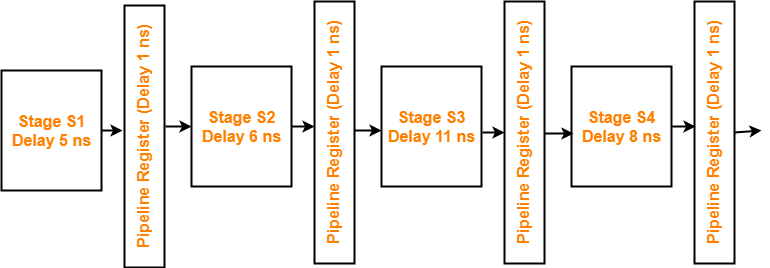What is the approximate speed up of the pipeline in steady state under ideal conditions when compared to the corresponding non-pipeline implementation?

1. 4.0
2. 2.5
3. 1.1
4. 3.0

## Solution-

### Non-Pipeline Execution Time-

Non-pipeline execution time for 1 instruction

= 5 ns + 6 ns + 11 ns + 8 ns

= 30 ns

### Cycle Time in Pipelined Processor-

Cycle time

= Maximum delay due to any stage + Delay due to its register

= Max { 5, 6, 11, 8 } + 1 ns

= 11 ns + 1 ns

= 12 ns

### Pipeline Execution Time-

Pipeline execution time

= 1 clock cycle

= 12 ns

### Speed Up-

Speed up

= Non-pipeline execution time / Pipeline execution time

= 30 ns / 12 ns

= 2.5

Thus, Option (B) is correct.

## Problem-08:

Consider a 4 stage pipeline processor. The number of cycles needed by the four instructions I1, I2, I3 and I4 in stages S1, S2, S3 and S4 is shown below-

 S1 S2 S3 S4 I1 2 1 1 1 I2 1 3 2 2 I3 2 1 1 3 I4 1 2 2 2

What is the number of cycles needed to execute the following loop?

for(i=1 to 2) { I1; I2; I3; I4; }

1. 16
2. 23
3. 28
4. 30

## Solution-

The phase-time diagram is-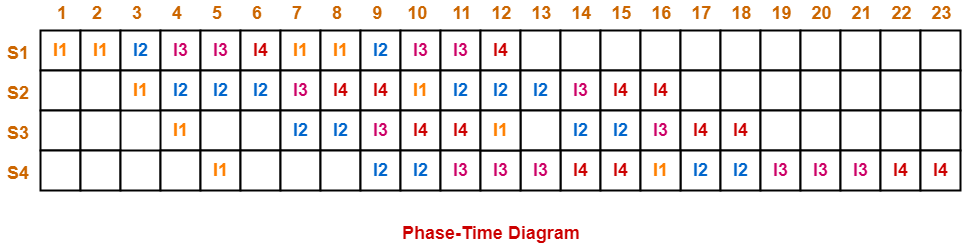From here, number of clock cycles required to execute the loop = 23 clock cycles.

Thus, Option (B) is correct.

## Problem-09:

Consider a pipelined processor with the following four stages-

IF : Instruction Fetch

ID : Instruction Decode and Operand Fetch

EX : Execute

WB : Write Back

The IF, ID and WB stages take one clock cycle each to complete the operation. The number of clock cycles for the EX stage depends on the instruction. The ADD and SUB instructions need 1 clock cycle and the MUL instruction need 3 clock cycles in the EX stage. Operand forwarding is used in the pipelined processor. What is the number of clock cycles taken to complete the following sequence of instructions?

ADD R2, R1, R0      R2 ← R0 + R1

MUL R4, R3, R2      R4 ← R3 + R2

SUB R6, R5, R4      R6 ← R5 + R4

1. 7
2. 8
3. 10
4. 14

## Solution-

The phase-time diagram is-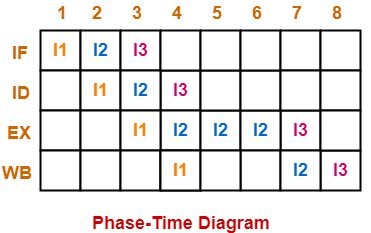From here, number of clock cycles required to execute the instructions = 8 clock cycles.

Thus, Option (B) is correct.

## Problem-10:

Consider the following procedures. Assume that the pipeline registers have zero latency.

P1 : 4 stage pipeline with stage latencies 1 ns, 2 ns, 2 ns, 1 ns

P2 : 4 stage pipeline with stage latencies 1 ns, 1.5 ns, 1.5 ns, 1.5 ns

P3 : 5 stage pipeline with stage latencies 0.5 ns, 1 ns, 1 ns, 0.6 ns, 1 ns

P4 : 5 stage pipeline with stage latencies 0.5 ns, 0.5 ns, 1 ns, 1 ns, 1.1 ns

Which procedure has the highest peak clock frequency?

1. P1
2. P2
3. P3
4. P4

## Solution-

It is given that pipeline registers have zero latency. Thus,

Cycle time

= Maximum delay due to any stage + Delay due to its register

= Maximum delay due to any stage

### For Processor P1:

Cycle time

= Max { 1 ns, 2 ns, 2 ns, 1 ns }

= 2 ns

Clock frequency

= 1 / Cycle time

= 1 / 2 ns

= 0.5 gigahertz

### For Processor P2:

Cycle time

= Max { 1 ns, 1.5 ns, 1.5 ns, 1.5 ns }

= 1.5 ns

Clock frequency

= 1 / Cycle time

= 1 / 1.5 ns

= 0.67 gigahertz

### For Processor P3:

Cycle time

= Max { 0.5 ns, 1 ns, 1 ns, 0.6 ns, 1 ns }

= 1 ns

Clock frequency

= 1 / Cycle time

= 1 / 1 ns

= 1 gigahertz

### For Processor P4:

Cycle time

= Max { 0.5 ns, 0.5 ns, 1 ns, 1 ns, 1.1 ns }

= 1.1 ns

Clock frequency

= 1 / Cycle time

= 1 / 1.1 ns

= 0.91 gigahertz

Clearly, Process P3 has the highest peak clock frequency.

Thus, Option (C) is correct.

## Problem-11:

Consider a 3 GHz (gigahertz) processor with a three-stage pipeline and stage latencies T1, T2 and T3 such that T1 = 3T2/4 = 2T3. If the longest pipeline stage is split into two pipeline stages of equal latency, the new frequency is ____ GHz, ignoring delays in the pipeline registers.

## Solution-

Let ‘t’ be the common multiple of each ratio, then-

• T1 = t
• T2 = 4t / 3
• T3 = t / 2

### Pipeline Cycle Time-

Pipeline cycle time

= Maximum delay due to any stage + Delay due to its register

= Max { t, 4t/3, t/2 } + 0

= 4t/3

### Frequency Of Pipeline-

Frequency

= 1 / Pipeline cycle time

= 1 / (4t / 3)

= 3 / 4t

Given frequency = 3 GHz. So,

3 / 4t = 3 GHz

4t = 1 ns

t = 0.25 ns

### Stage Latencies Of Pipeline-

Stage latency of different stages are-

• Latency of stage-01 = 0.25 ns
• Latency of stage-02 = 0.33 ns
• Latency of stage-03 = 0.125 ns

### Splitting The Pipeline-

The stage with longest latency i.e. stage-02 is split up into 4 stages.

After splitting, the latency of different stages are-

• Latency of stage-01 = 0.25 ns
• Latency of stage-02 = 0.165 ns
• Latency of stage-03 = 0.165 ns
• Latency of stage-04 = 0.125 ns

### Splitted Pipeline Cycle Time-

Splitted pipeline cycle time

= Maximum delay due to any stage + Delay due to its register

= Max { 0.25, 0.165, 0.165, 0.125 } + 0

= 0.25 ns

### Frequency Of Splitted Pipeline-

Frequency

= 1 / splitted pipeline cycle time

= 1 / 0.25 ns

= 4 GHz

Thus, new frequency = 4 GHz.

Get more notes and other study material of Computer Organization and Architecture.

Watch video lectures by visiting our YouTube channel LearnVidFun.

## Instruction Pipelining-

Before you go through this article, make sure that you have gone through the previous article on Instruction Pipelining.

In instruction pipelining,

• A form of parallelism called as instruction level parallelism is implemented.
• Multiple instructions execute simultaneously.
• The efficiency of pipelined execution is more than that of non-pipelined execution.

## Performance of Pipelined Execution-

The following parameters serve as criterion to estimate the performance of pipelined execution-

• Speed Up
• Efficiency
• Throughput

## 1. Speed Up-

It gives an idea of “how much faster” the pipelined execution is as compared to non-pipelined execution.

It is calculated as-## 2. Efficiency-

The efficiency of pipelined execution is calculated as-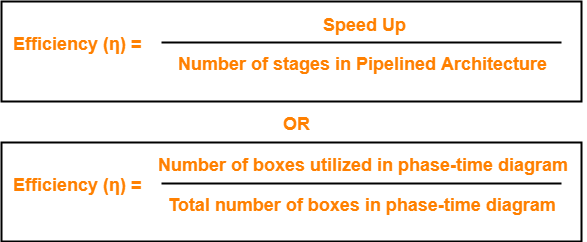## 3. Throughput-

Throughput is defined as number of instructions executed per unit time.

It is calculated as-## Calculation of Important Parameters-

Let us learn how to calculate certain important parameters of pipelined architecture.

Consider-

• A pipelined architecture consisting of k-stage pipeline
• Total number of instructions to be executed = n

## Point-01: Calculating Cycle Time-

In pipelined architecture,

• There is a global clock that synchronizes the working of all the stages.
• Frequency of the clock is set such that all the stages are synchronized.
• At the beginning of each clock cycle, each stage reads the data from its register and process it.
• Cycle time is the value of one clock cycle.

There are two cases possible-

### Case-01: All the stages offer same delay-

If all the stages offer same delay, then-

Cycle time = Delay offered by one stage including the delay due to its register

### Case-02: All the stages do not offer same delay-

If all the stages do not offer same delay, then-

Cycle time = Maximum delay offered by any stage including the delay due to its register

## Point-02: Calculating Frequency Of Clock-

Frequency of the clock (f) = 1 / Cycle time

## Point-03: Calculating Non-Pipelined Execution Time-

In non-pipelined architecture,

• The instructions execute one after the other.
• The execution of a new instruction begins only after the previous instruction has executed completely.
• So, number of clock cycles taken by each instruction = k clock cycles

Thus,

Non-pipelined execution time

= Total number of instructions x Time taken to execute one instruction

= n x k clock cycles

## Point-04: Calculating Pipelined Execution Time-

In pipelined architecture,

• Multiple instructions execute parallely.
• Number of clock cycles taken by the first instruction = k clock cycles
• After first instruction has completely executed, one instruction comes out per clock cycle.
• So, number of clock cycles taken by each remaining instruction = 1 clock cycle

Thus,

Pipelined execution time

= Time taken to execute first instruction + Time taken to execute remaining instructions

= 1 x k clock cycles + (n-1) x 1 clock cycle

= (k + n – 1) clock cycles

## Point-04: Calculating Speed Up-

Speed up

= Non-pipelined execution time / Pipelined execution time

= n x k clock cycles / (k + n – 1) clock cycles

= n x k / (k + n – 1)

= n x k / n + (k – 1)

= k / { 1 + (k – 1)/n }

• For very large number of instructions, n→∞. Thus, speed up = k.
• Practically, total number of instructions never tend to infinity.
• Therefore, speed up is always less than number of stages in pipeline.

## Important Notes-

### Note-01:

• The aim of pipelined architecture is to execute one complete instruction in one clock cycle.
• In other words, the aim of pipelining is to maintain CPI ≅ 1.
• Practically, it is not possible to achieve CPI ≅ 1 due to delays that get introduced due to registers.
• Ideally, a pipelined architecture executes one complete instruction per clock cycle (CPI=1).

### Note-02:

• The maximum speed up that can be achieved is always equal to the number of stages.
• This is achieved when efficiency becomes 100%.
• Practically, efficiency is always less than 100%.
• Therefore speed up is always less than number of stages in pipelined architecture.

### Note-03:

Under ideal conditions,

• One complete instruction is executed per clock cycle i.e. CPI = 1.
• Speed up = Number of stages in pipelined architecture

### Note-04:

• Experiments show that 5 stage pipelined processor gives the best performance.

### Note-05:

In case only one instruction has to be executed, then-

• Non-pipelined execution gives better performance than pipelined execution.
• This is because delays are introduced due to registers in pipelined architecture.
• Thus, time taken to execute one instruction in non-pipelined architecture is less.

### Note-06:

High efficiency of pipelined processor is achieved when-

• All the stages are of equal duration.
• There are no conditional branch instructions.
• There are no interrupts.
• There are no register and memory conflicts.

Performance degrades in absence of these conditions.

To gain better understanding about Pipelining in Computer Architecture,

Watch this Video Lecture

Next Article- Practice Problems On Pipelining

Get more notes and other study material of Computer Organization and Architecture.

Watch video lectures by visiting our YouTube channel LearnVidFun.

## Introduction-

• A program consists of several number of instructions.
• These instructions may be executed in the following two ways-1. Non-Pipelined Execution
2. Pipelined Execution

## 1. Non-Pipelined Execution-

In non-pipelined architecture,

• All the instructions of a program are executed sequentially one after the other.
• A new instruction executes only after the previous instruction has executed completely.
• This style of executing the instructions is highly inefficient.

## Example-

Consider a program consisting of three instructions.

In a non-pipelined architecture, these instructions execute one after the other as-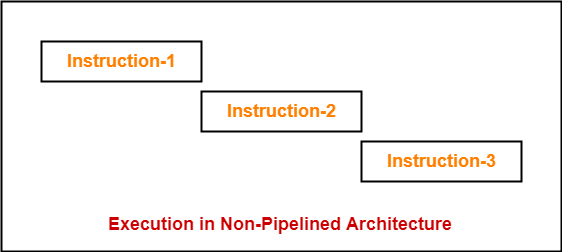If time taken for executing one instruction = t, then-

Time taken for executing ‘n’ instructions = n x t

## 2. Pipelined Execution-

In pipelined architecture,

• Multiple instructions are executed parallely.
• This style of executing the instructions is highly efficient.

Now, let us discuss instruction pipelining in detail.

## Instruction Pipelining-

 Instruction pipelining is a technique that implements a form of parallelism called as instruction level parallelism within a single processor.

• A pipelined processor does not wait until the previous instruction has executed completely.
• Rather, it fetches the next instruction and begins its execution.

## Pipelined Architecture-

In pipelined architecture,

• The hardware of the CPU is split up into several functional units.
• Each functional unit performs a dedicated task.
• The number of functional units may vary from processor to processor.
• These functional units are called as stages of the pipeline.
• Control unit manages all the stages using control signals.
• There is a register associated with each stage that holds the data.
• There is a global clock that synchronizes the working of all the stages.
• At the beginning of each clock cycle, each stage takes the input from its register.
• Each stage then processes the data and feed its output to the register of the next stage.

## Four-Stage Pipeline-

In four stage pipelined architecture, the execution of each instruction is completed in following 4 stages-

1. Instruction fetch (IF)
2. Instruction decode (ID)
3. Instruction Execute (IE)
4. Write back (WB)

To implement four stage pipeline,

• The hardware of the CPU is divided into four functional units.
• Each functional unit performs a dedicated task.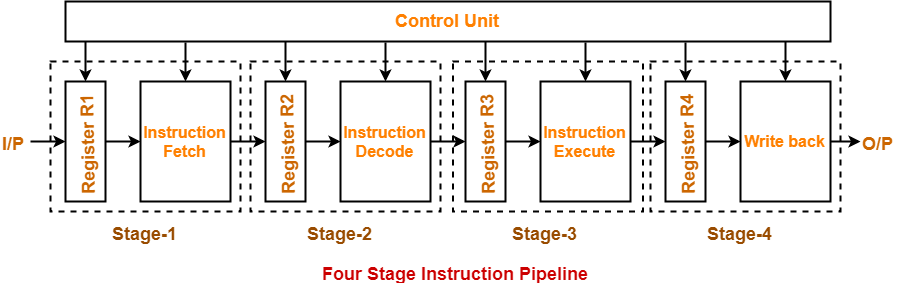### Stage-01:

At stage-01,

• First functional unit performs instruction fetch.
• It fetches the instruction to be executed.

### Stage-02:

At stage-02,

• Second functional unit performs instruction decode.
• It decodes the instruction to be executed.

### Stage-03:

At stage-03,

• Third functional unit performs instruction execution.
• It executes the instruction.

### Stage-04:

At stage-04,

• Fourth functional unit performs write back.
• It writes back the result so obtained after executing the instruction.

## Execution-

In pipelined architecture,

• Instructions of the program execute parallely.
• When one instruction goes from nth stage to (n+1)th stage, another instruction goes from (n-1)th stage to nth stage.

## Phase-Time Diagram-

• Phase-time diagram shows the execution of instructions in the pipelined architecture.
• The following diagram shows the execution of three instructions in four stage pipeline architecture.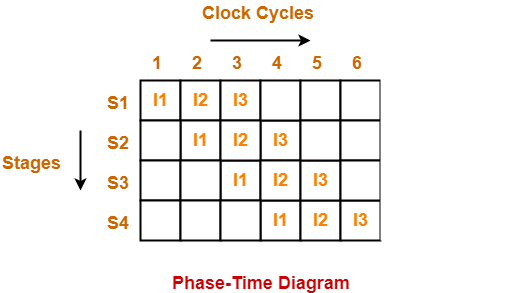Time taken to execute three instructions in four stage pipelined architecture = 6 clock cycles.

### NOTE-

In non-pipelined architecture,

Time taken to execute three instructions would be

= 3 x Time taken to execute one instruction

= 3 x 4 clock cycles

= 12 clock cycles

Clearly, pipelined execution of instructions is far more efficient than non-pipelined execution.

To gain better understanding about Pipelining in Computer Architecture,

Watch this Video Lecture

Next Article- Instruction Pipeline | Formulas

Get more notes and other study material of Computer Organization and Architecture.

Watch video lectures by visiting our YouTube channel LearnVidFun.